##### Corporate Finance For Dummies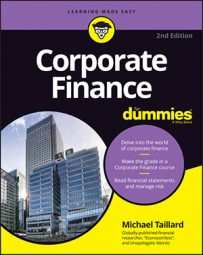Corporate debt is a big deal. In fact, it’s such a big deal that companies value their capital structure based on how effectively they manage debt. Why is debt so important as compared to, say, equity?

First, a company can have more debt than assets. Unlike equity, where the maximum equity is the value of all the company’s assets, debt can surpass assets. Second, debt incurs interest that needs to be paid off; equity doesn’t. Third, as far as the capital structure goes, using debt as a measure usually provides important information about equity as well.

## Times interest earned

When evaluating a company’s debt structure, you need to know whether a company can pay the interest it owes on the debt it has incurred. To find out, you can use times interest earned, which looks like this: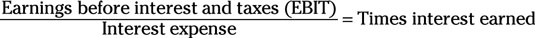To use this equation, follow these steps:

1. Find EBIT near the middle or bottom of the income statement and interest expense somewhere below that.

2. Divide EBIT by interest expense to calculate the times interest earned.

A low times interest earned may mean the company is at risk of defaulting on its debt obligations, which is a bad sign for its level of earnings. But a very high interest earned may mean the company isn’t fully utilizing its available capital and could possibly generate additional sales by acquiring more debt to expand its production capacity, particularly if earnings have reached a plateau with the company already producing at full capacity.

## Fixed charge coverage

Interest is only one form of fixed charge that a company can default on. Leases are another particularly common form, but there are many others, as well. To determine whether a company will default on any of these charges, you can use the metric called fixed charge coverage. Although this equation has a few variations, this one is the most common: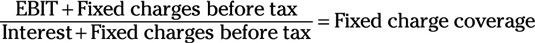Follow these steps to use this equation:

1. Find EBIT, fixed charges before tax, and interest expense on the income statement.

2. Add EBIT and fixed charges before tax.

3. Add interest and fixed charges before tax.

4. Divide the answer from Step 2 by the answer from Step 3 to calculate the fixed charge coverage.

This metric is extremely similar to times interest earned, but adding the same value (fixed charges before tax) to both the top and bottom of the equation is guaranteed to change the end value. Fixed charge coverage is particularly important for companies that have a high portion of fixed charges other than interest.

## Debt ratio

A company with an excessive amount of debt is at very serious risk of default, so it’s no wonder that a company’s debt ratio is important to a number of its constituents. Lenders like to know a company’s debt ratio because they want to be reassured that they’ll get their money back — even if the company goes out of business.

Investors like to know a company’s debt ratio because they want to be reassured that they’ll be owning a company that’s worth the value of their investment. Companies themselves like to know their debt ratio to determine whether they’re at risk of defaulting on that debt. You can determine all this and more with the help of one little equation: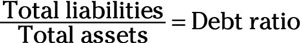Here’s how to use this equation:

1. Find total liabilities in the liabilities portion of the balance sheet and total assets in the assets portion.

2. Divide total liabilities by total assets to get the debt ratio.

The debt ratio tells you what percentage of a company’s total assets were funded by incurring debt. A debt ratio of more than 1 means the company actually has more debt than the company is worth. A debt ratio of less than 1 means the company has more assets than the debt it owes. A debt ratio anywhere near 1 is a bad position to be in, much less a ratio higher than 1.

## Debt to equity ratio

When measuring a company’s capital structure, you need to calculate the debt to equity ratio, which tells you the ratio that liabilities compose of a company’s funding compared to equity. Here’s what this equation looks like: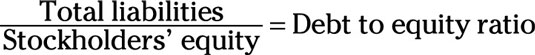To use this equation, follow these steps:

1. Find total liabilities in the liabilities portion of the balance sheet and stockholders’ equity in the equity portion (nothing like stating the obvious!).

2. Divide total liabilities by stockholders’ equity to find the debt to equity ratio.

A high debt to equity ratio can mean two different things: If a company also has a low times interest earned, then it was probably a bit too reliant on funding operations with debt and will have a hard time paying its interest. If the company also has a very high times interest earned, then it was likely incurring debt to generate funding beyond what it could earn selling debt to generate sales.

As long as the extra ratio of debt increases a company’s times interest earned, then the differential in earnings will increase the value of equity, balancing out the debt to equity ratio in the long run.

## Debt to tangible net worth

If a company were to default on its debt, get bought by an investor who intends to sell off the assets, or otherwise go out of business, everyone involved would likely want to know the value of the physical assets owned by the company. You can’t sell off intellectual property when liquidating a company, so you need to use the ratio called debt to tangible net worth: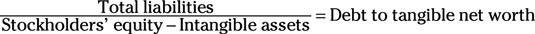Follow these steps to use this equation:

1. Find total liabilities in the liabilities portion of the balance sheet, stockholders’ equity in the equity portion of the income statement, and intangible assets in the assets portion of the income statement.

2. Subtract the value of intangible assets from the value of stockholders’ equity.

3. Divide total liabilities from the answer from Step 2 to find the debt to tangible net worth ratio.

If the ratio is greater than 1, the company has more debt than it could pay off by liquidating all its assets. If the ratio is less than 1, the company could pay off all its debt by liquidating its assets and still have some left over. In the 1980s, many investors purchased companies with very low debt to tangible net worth ratios and made profits by selling off the assets.

## Operating cash flows to total debt

When possible, companies prefer not to have to sell off their assets in order to pay their debt. After all, having to do so is usually a sign that the company is in big trouble. To measure a company’s ability to pay off debt without actually selling off its assets, you can use the operating cash flows to total debt ratio: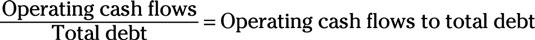Here’s how to use this ratio:

1. Find operating cash flows on the statement of cash flows and total debt in the debt portion of the balance sheet.

2. Divide operating cash flows by total debt to get the operating cash flows to debt ratio.

A high ratio means the company is probably able to pay off its debts by using cash flows. A low ratio means the company may end up having to sell stuff off to pay its debts.

## Equity multiplier

The equity multiplier measures the ratio of a company’s assets that stockholders own: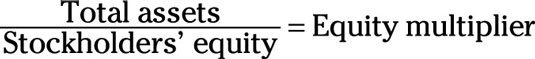To put this equation to use, follow these steps:

1. Find total assets in the assets portion of the balance sheet and stockholders’ equity in the equity portion.

2. Divide total assets by stockholders’ equity to get the equity multiplier.

A ratio of 1 means that all the company’s assets are funded through equity and that the company has no debt. In other words, the company has one dollar’s worth of assets for every dollar’s worth of equity. Even if the company increases in value, unless it incurs debt, that increased value will go toward increasing stockholders’ equity.

On the other hand, a ratio of less than one means that the company used debt to fund its activities.